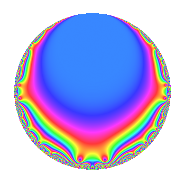# Properties

 Label 62.2.c.aLevel 62 Weight 2 Character orbit 62.c Analytic conductor 0.495 Analytic rank 0 Dimension 2 CM No Inner twists 2

# Related objects

## Newspace parameters

 Level: $$N$$ = $$62 = 2 \cdot 31$$ Weight: $$k$$ = $$2$$ Character orbit: $$[\chi]$$ = 62.c (of order $$3$$ and degree $$2$$)

## Newform invariants

 Self dual: No Analytic conductor: $$0.495072492532$$ Analytic rank: $$0$$ Dimension: $$2$$ Coefficient field: $$\Q(\sqrt{-3})$$ Coefficient ring: $$\Z[a_1, a_2, a_3]$$ Coefficient ring index: $$1$$ Sato-Tate group: $\mathrm{SU}(2)[C_{3}]$

## $q$-expansion

Coefficients of the $$q$$-expansion are expressed in terms of a primitive root of unity $$\zeta_{6}$$. We also show the integral $$q$$-expansion of the trace form.

 $$f(q)$$ $$=$$ $$q - q^{2} + ( -1 + \zeta_{6} ) q^{3} + q^{4} + 3 \zeta_{6} q^{5} + ( 1 - \zeta_{6} ) q^{6} + ( 1 - \zeta_{6} ) q^{7} - q^{8} + 2 \zeta_{6} q^{9} +O(q^{10})$$ $$q - q^{2} + ( -1 + \zeta_{6} ) q^{3} + q^{4} + 3 \zeta_{6} q^{5} + ( 1 - \zeta_{6} ) q^{6} + ( 1 - \zeta_{6} ) q^{7} - q^{8} + 2 \zeta_{6} q^{9} -3 \zeta_{6} q^{10} -3 \zeta_{6} q^{11} + ( -1 + \zeta_{6} ) q^{12} -5 \zeta_{6} q^{13} + ( -1 + \zeta_{6} ) q^{14} -3 q^{15} + q^{16} + ( -3 + 3 \zeta_{6} ) q^{17} -2 \zeta_{6} q^{18} + ( 7 - 7 \zeta_{6} ) q^{19} + 3 \zeta_{6} q^{20} + \zeta_{6} q^{21} + 3 \zeta_{6} q^{22} + ( 1 - \zeta_{6} ) q^{24} + ( -4 + 4 \zeta_{6} ) q^{25} + 5 \zeta_{6} q^{26} -5 q^{27} + ( 1 - \zeta_{6} ) q^{28} + 6 q^{29} + 3 q^{30} + ( -5 + 6 \zeta_{6} ) q^{31} - q^{32} + 3 q^{33} + ( 3 - 3 \zeta_{6} ) q^{34} + 3 q^{35} + 2 \zeta_{6} q^{36} + ( 7 - 7 \zeta_{6} ) q^{37} + ( -7 + 7 \zeta_{6} ) q^{38} + 5 q^{39} -3 \zeta_{6} q^{40} -3 \zeta_{6} q^{41} -\zeta_{6} q^{42} + ( -5 + 5 \zeta_{6} ) q^{43} -3 \zeta_{6} q^{44} + ( -6 + 6 \zeta_{6} ) q^{45} -12 q^{47} + ( -1 + \zeta_{6} ) q^{48} + 6 \zeta_{6} q^{49} + ( 4 - 4 \zeta_{6} ) q^{50} -3 \zeta_{6} q^{51} -5 \zeta_{6} q^{52} -9 \zeta_{6} q^{53} + 5 q^{54} + ( 9 - 9 \zeta_{6} ) q^{55} + ( -1 + \zeta_{6} ) q^{56} + 7 \zeta_{6} q^{57} -6 q^{58} + ( 3 - 3 \zeta_{6} ) q^{59} -3 q^{60} -10 q^{61} + ( 5 - 6 \zeta_{6} ) q^{62} + 2 q^{63} + q^{64} + ( 15 - 15 \zeta_{6} ) q^{65} -3 q^{66} + 13 \zeta_{6} q^{67} + ( -3 + 3 \zeta_{6} ) q^{68} -3 q^{70} + 3 \zeta_{6} q^{71} -2 \zeta_{6} q^{72} + 13 \zeta_{6} q^{73} + ( -7 + 7 \zeta_{6} ) q^{74} -4 \zeta_{6} q^{75} + ( 7 - 7 \zeta_{6} ) q^{76} -3 q^{77} -5 q^{78} + ( 1 - \zeta_{6} ) q^{79} + 3 \zeta_{6} q^{80} + ( -1 + \zeta_{6} ) q^{81} + 3 \zeta_{6} q^{82} + 9 \zeta_{6} q^{83} + \zeta_{6} q^{84} -9 q^{85} + ( 5 - 5 \zeta_{6} ) q^{86} + ( -6 + 6 \zeta_{6} ) q^{87} + 3 \zeta_{6} q^{88} + 6 q^{89} + ( 6 - 6 \zeta_{6} ) q^{90} -5 q^{91} + ( -1 - 5 \zeta_{6} ) q^{93} + 12 q^{94} + 21 q^{95} + ( 1 - \zeta_{6} ) q^{96} + 2 q^{97} -6 \zeta_{6} q^{98} + ( 6 - 6 \zeta_{6} ) q^{99} +O(q^{100})$$ $$\operatorname{Tr}(f)(q)$$ $$=$$ $$2q - 2q^{2} - q^{3} + 2q^{4} + 3q^{5} + q^{6} + q^{7} - 2q^{8} + 2q^{9} + O(q^{10})$$ $$2q - 2q^{2} - q^{3} + 2q^{4} + 3q^{5} + q^{6} + q^{7} - 2q^{8} + 2q^{9} - 3q^{10} - 3q^{11} - q^{12} - 5q^{13} - q^{14} - 6q^{15} + 2q^{16} - 3q^{17} - 2q^{18} + 7q^{19} + 3q^{20} + q^{21} + 3q^{22} + q^{24} - 4q^{25} + 5q^{26} - 10q^{27} + q^{28} + 12q^{29} + 6q^{30} - 4q^{31} - 2q^{32} + 6q^{33} + 3q^{34} + 6q^{35} + 2q^{36} + 7q^{37} - 7q^{38} + 10q^{39} - 3q^{40} - 3q^{41} - q^{42} - 5q^{43} - 3q^{44} - 6q^{45} - 24q^{47} - q^{48} + 6q^{49} + 4q^{50} - 3q^{51} - 5q^{52} - 9q^{53} + 10q^{54} + 9q^{55} - q^{56} + 7q^{57} - 12q^{58} + 3q^{59} - 6q^{60} - 20q^{61} + 4q^{62} + 4q^{63} + 2q^{64} + 15q^{65} - 6q^{66} + 13q^{67} - 3q^{68} - 6q^{70} + 3q^{71} - 2q^{72} + 13q^{73} - 7q^{74} - 4q^{75} + 7q^{76} - 6q^{77} - 10q^{78} + q^{79} + 3q^{80} - q^{81} + 3q^{82} + 9q^{83} + q^{84} - 18q^{85} + 5q^{86} - 6q^{87} + 3q^{88} + 12q^{89} + 6q^{90} - 10q^{91} - 7q^{93} + 24q^{94} + 42q^{95} + q^{96} + 4q^{97} - 6q^{98} + 6q^{99} + O(q^{100})$$

## Character Values

We give the values of $$\chi$$ on generators for $$\left(\mathbb{Z}/62\mathbb{Z}\right)^\times$$.

 $$n$$ $$3$$ $$\chi(n)$$ $$-\zeta_{6}$$

## Embeddings

For each embedding $$\iota_m$$ of the coefficient field, the values $$\iota_m(a_n)$$ are shown below.

For more information on an embedded modular form you can click on its label.

Label $$\iota_m(\nu)$$ $$a_{2}$$ $$a_{3}$$ $$a_{4}$$ $$a_{5}$$ $$a_{6}$$ $$a_{7}$$ $$a_{8}$$ $$a_{9}$$ $$a_{10}$$
5.1
 0.5 + 0.866025i 0.5 − 0.866025i
−1.00000 −0.500000 + 0.866025i 1.00000 1.50000 + 2.59808i 0.500000 0.866025i 0.500000 0.866025i −1.00000 1.00000 + 1.73205i −1.50000 2.59808i
25.1 −1.00000 −0.500000 0.866025i 1.00000 1.50000 2.59808i 0.500000 + 0.866025i 0.500000 + 0.866025i −1.00000 1.00000 1.73205i −1.50000 + 2.59808i
 $$n$$: e.g. 2-40 or 990-1000 Significant digits: Format: Complex embeddings Normalized embeddings Satake parameters Satake angles

## Inner twists

Char. orbit Parity Mult. Self Twist Proved
1.a Even 1 trivial yes
31.c Even 1 yes

## Hecke kernels

This newform can be constructed as the kernel of the linear operator $$T_{3}^{2} + T_{3} + 1$$ acting on $$S_{2}^{\mathrm{new}}(62, [\chi])$$.